# Fundamental Trigonometric 14 3 Identities 14 3 Trigonometric

• Slides: 22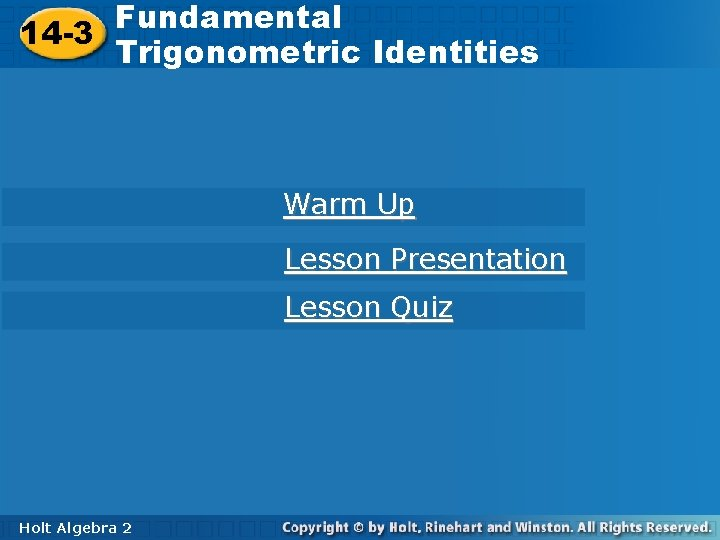Fundamental. Trigonometric 14 -3 Identities 14 -3 Trigonometric Identities Warm Up Lesson Presentation Lesson Quiz Holt Algebra 22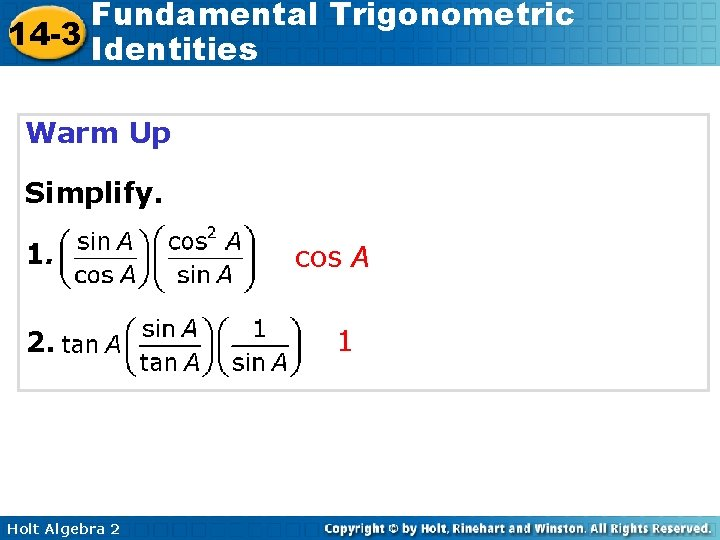Fundamental Trigonometric 14 -3 Identities Warm Up Simplify. 1. 2. Holt Algebra 2 cos A 1Fundamental Trigonometric 14 -3 Identities Objective Use fundamental trigonometric identities to simplify and rewrite expressions and to verify other identities. Holt Algebra 2Fundamental Trigonometric 14 -3 Identities You can use trigonometric identities to simplify trigonometric expressions. Recall that an identity is a mathematical statement that is true for all values of the variables for which the statement is defined. Holt Algebra 2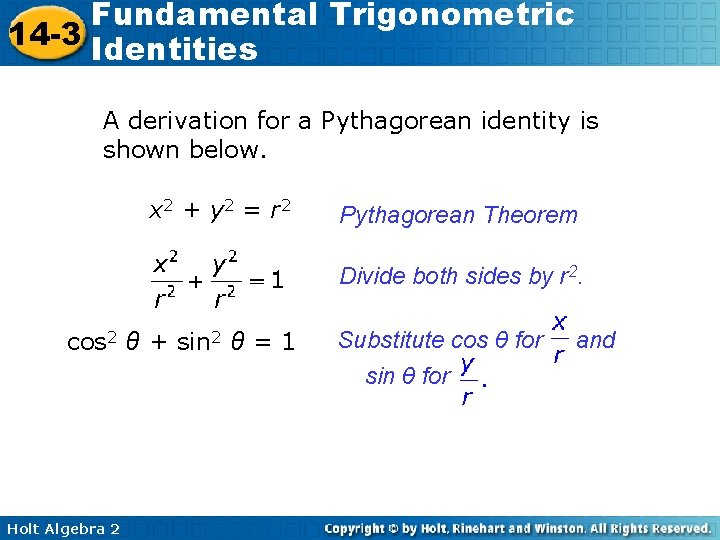Fundamental Trigonometric 14 -3 Identities A derivation for a Pythagorean identity is shown below. x 2 + y 2 = r 2 Pythagorean Theorem Divide both sides by r 2. cos 2 θ + sin 2 θ = 1 Holt Algebra 2 Substitute cos θ for sin θ for andFundamental Trigonometric 14 -3 Identities To prove that an equation is an identity, alter one side of the equation until it is the same as the other side. Justify your steps by using the fundamental identities. Holt Algebra 2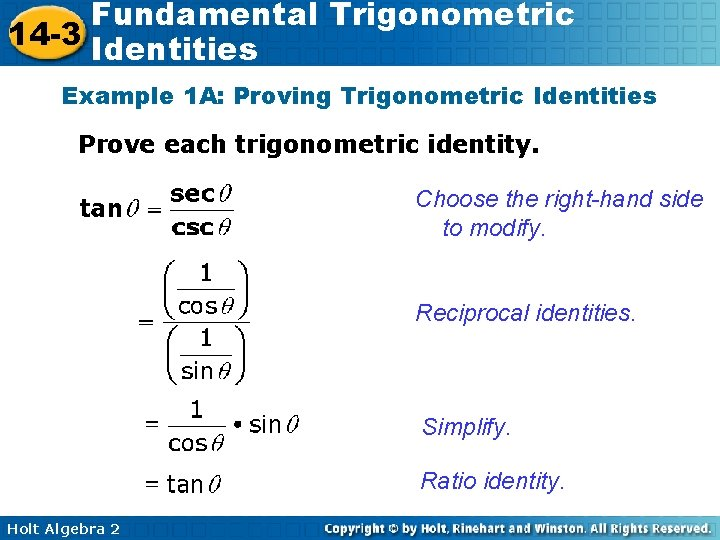Fundamental Trigonometric 14 -3 Identities Example 1 A: Proving Trigonometric Identities Prove each trigonometric identity. Choose the right-hand side to modify. Reciprocal identities. Simplify. Ratio identity. Holt Algebra 2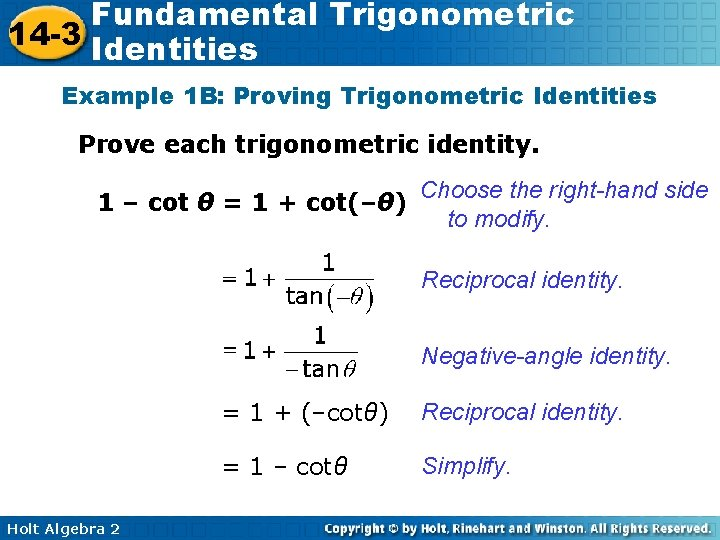Fundamental Trigonometric 14 -3 Identities Example 1 B: Proving Trigonometric Identities Prove each trigonometric identity. 1 – cot θ = 1 + cot(–θ) Choose the right-hand side to modify. Reciprocal identity. Negative-angle identity. Holt Algebra 2 = 1 + (–cotθ) Reciprocal identity. = 1 – cotθ Simplify.Fundamental Trigonometric 14 -3 Identities Helpful Hint You may start with either side of the given equation. It is often easier to begin with the more complicated side and simplify it to match the simpler side. Holt Algebra 2Fundamental Trigonometric 14 -3 Identities Check It Out! Example 1 a Prove each trigonometric identity. sin θ cot θ = cos θ = cos θ Holt Algebra 2 Choose the left-hand side to modify. Ratio identity. Simplify.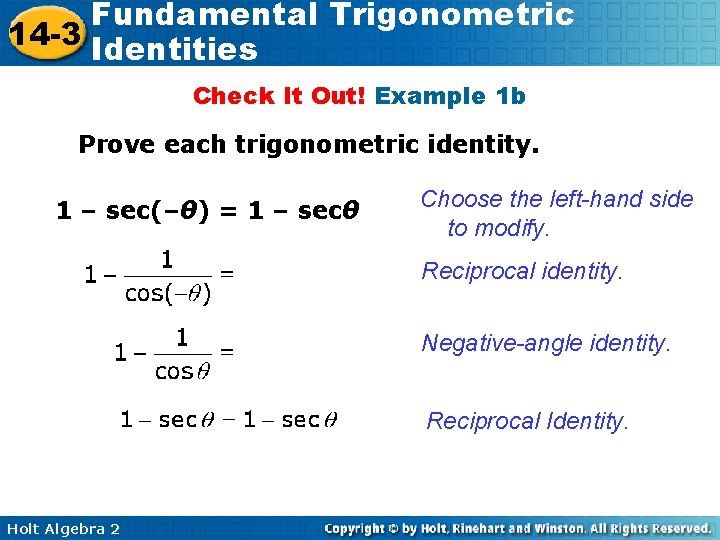Fundamental Trigonometric 14 -3 Identities Check It Out! Example 1 b Prove each trigonometric identity. 1 – sec(–θ) = 1 – secθ Choose the left-hand side to modify. Reciprocal identity. Negative-angle identity. Reciprocal Identity. Holt Algebra 2Fundamental Trigonometric 14 -3 Identities You can use the fundamental trigonometric identities to simplify expressions. Helpful Hint If you get stuck, try converting all of the trigonometric functions to sine and cosine functions. Holt Algebra 2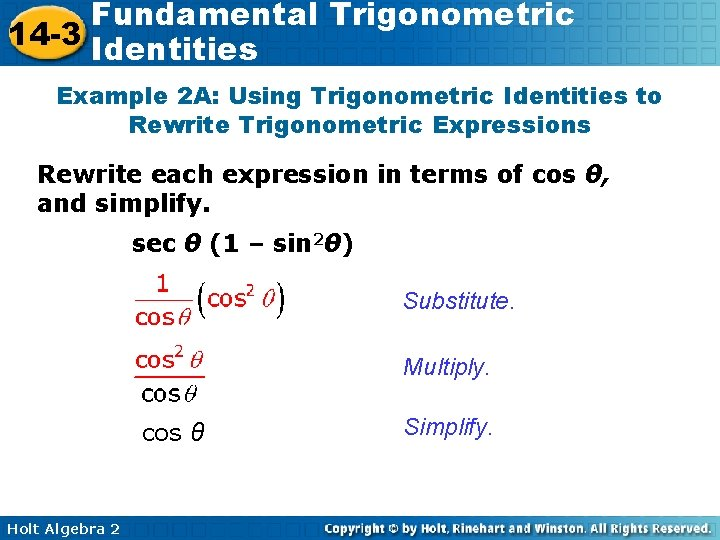Fundamental Trigonometric 14 -3 Identities Example 2 A: Using Trigonometric Identities to Rewrite Trigonometric Expressions Rewrite each expression in terms of cos θ, and simplify. sec θ (1 – sin 2θ) Substitute. Multiply. cos θ Holt Algebra 2 Simplify.Fundamental Trigonometric 14 -3 Identities Example 2 B: Using Trigonometric Identities to Rewrite Trigonometric Expressions Rewrite each expression in terms of sin θ, cos θ, and simplify. sinθ cosθ(tanθ + cotθ) Substitute. Multiply. sin 2θ + cos 2θ 1 Holt Algebra 2 Simplify. Pythagorean identity.Fundamental Trigonometric 14 -3 Identities Check It Out! Example 2 a Rewrite each expression in terms of sin θ, and simplify. Pythagorean identity. Factor the difference of two squares. Simplify. Holt Algebra 2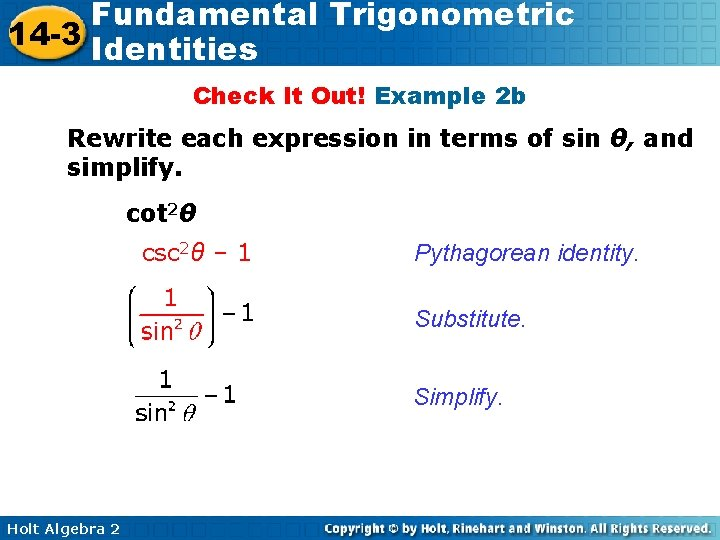Fundamental Trigonometric 14 -3 Identities Check It Out! Example 2 b Rewrite each expression in terms of sin θ, and simplify. cot 2θ csc 2θ – 1 Pythagorean identity. Substitute. Simplify. Holt Algebra 2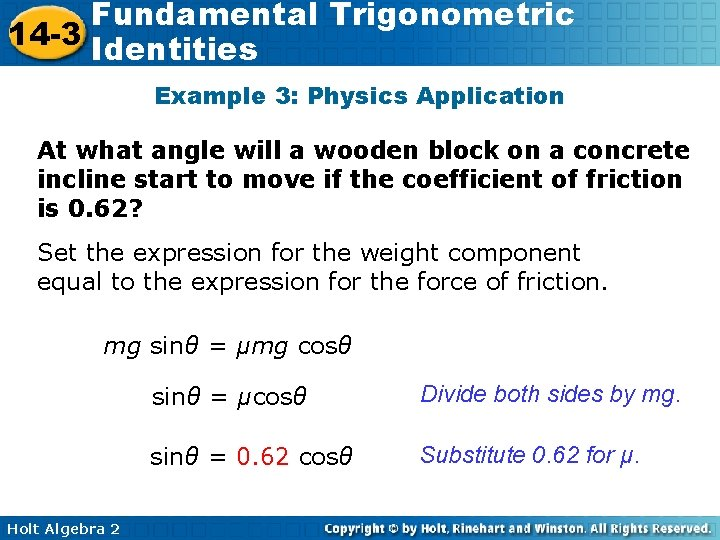Fundamental Trigonometric 14 -3 Identities Example 3: Physics Application At what angle will a wooden block on a concrete incline start to move if the coefficient of friction is 0. 62? Set the expression for the weight component equal to the expression for the force of friction. mg sinθ = μmg cosθ Holt Algebra 2 sinθ = μcosθ Divide both sides by mg. sinθ = 0. 62 cosθ Substitute 0. 62 for μ.Fundamental Trigonometric 14 -3 Identities Example 3 Continued Divide both sides by cos θ. tanθ = 0. 62 θ = 32° Ratio identity. Evaluate inverse tangent. The wooden block will start to move when the concrete incline is raised to an angle of about 32°. Holt Algebra 2Fundamental Trigonometric 14 -3 Identities Check It Out! Example 3 Use the equation mg sinθ = μmg cosθ to determine the angle at which a waxed wood block on a wood incline with μ = 0. 4 begins to slide. Set the expression for the weight component equal to the expression for the force of friction. mg sinθ = μmg cosθ Holt Algebra 2 sinθ = μcosθ Divide both sides by mg. sinθ = 0. 4 cosθ Substitute 0. 4 for μ.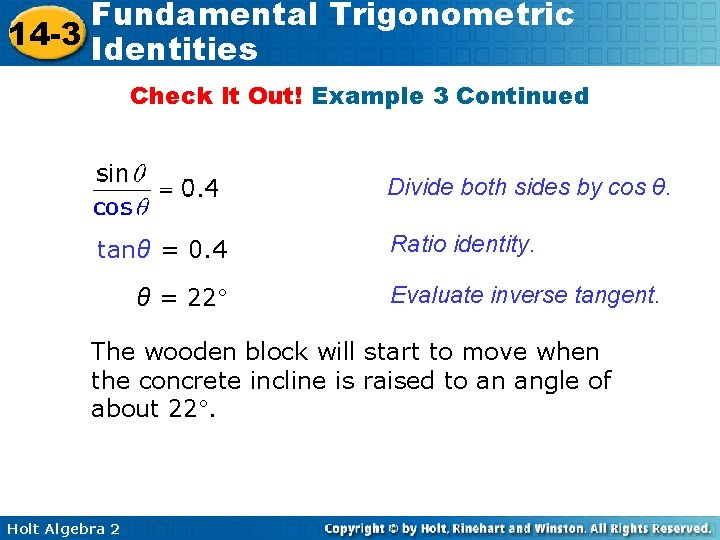Fundamental Trigonometric 14 -3 Identities Check It Out! Example 3 Continued Divide both sides by cos θ. tanθ = 0. 4 θ = 22° Ratio identity. Evaluate inverse tangent. The wooden block will start to move when the concrete incline is raised to an angle of about 22°. Holt Algebra 2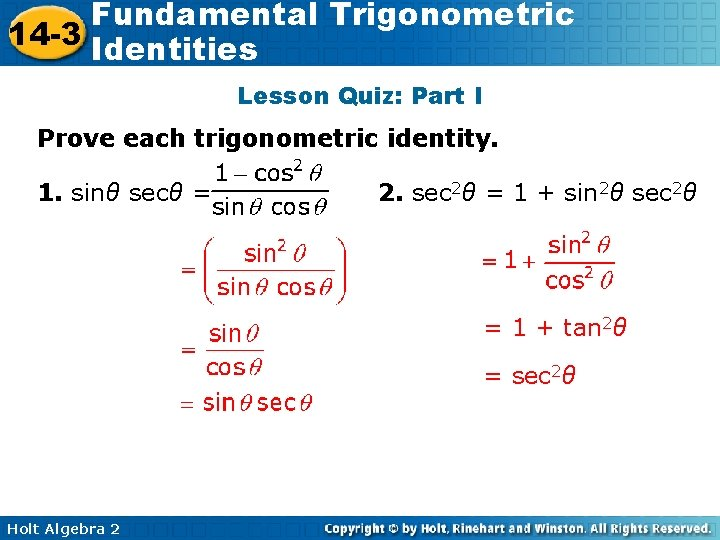Fundamental Trigonometric 14 -3 Identities Lesson Quiz: Part I Prove each trigonometric identity. 1. sinθ secθ = 2. sec 2θ = 1 + sin 2θ sec 2θ = 1 + tan 2θ = sec 2θ Holt Algebra 2Fundamental Trigonometric 14 -3 Identities Lesson Quiz: Part II Rewrite each expression in terms of cos θ, and simplify. 3. sin 2θ cot 2θ secθ cosθ 4. 2 cosθ Holt Algebra 2